# SuiteCRM Calculation Field

Create business logic for field calculations without needing to write custom code. You can select calculated field and create the formula that is automatically updated based on calculation you provide.

#### Documentation

TechEsperto Solutions LLC Website: https://www.techesperto.com/ Email: info@techesperto.com

We are a Global Turnkey IT Solution and Technology Service Provider committed towards delivering innovative and quality solutions. With deep industry, business process and technology expertise, broad global resources and a proven track record, we collaborate with clients to help them become high-performance organizations.

### SuiteCRM Calculation Field

Here we allow Administrators to create business logic for field/manual calculations without needing to write custom code. You can select calculated field and create the formula that is automatically updated based on calculation you provide.

### 1.Key Features

• You can give a field in your formula for dynamic calculations
• Available Functions : Add, Subtract, Multiply, Divide, String Length, Concatenation, Log(Logarithm), Ln(Natural Log), Absolute, Average, Power, Date Difference, Percentage, Mod(Modulo), Minimum, Negate, Floor, Ceil ,Add hours,Add day,Add week,Add month,Add year,Sub hours,Sub days,Sub week,Sub month,Sub year,Diff days,Diff hour,Diff minute,Diff month,Week Diff,Diff year Count Relationship ,Sum of Relate Fields
• A rich conditional support we provide: you can make a big calculation here, a nested formula
• You can insert manual value or Field or both together to create formula. For e.g. add(field1,200,sub(100,field2))
• You can use calculated field also to make further formula

### 2.Calculated Field Functions syntax

• Subtract: sub(Number field1,Number field2): Returns field1 minus field2, For e.g: sub(2,1)=1
• Multiply: mul(Number field,...): Returns the multiplication of given numbers in field, For e.g: mul(2,3,-4)=-24
• Divide: div(Number numerator_field,Number denominator_field): Returns the numerator_field divided by denominator_field. For e.g: div(10,2)=5
• String Length: strlen(String field): Returns the total length of string. For e.g:strlen('Hello World')=11
• Concatenation: concat(String field,...): Returns the string that results from concatenating the arguments. For e.g: concat(Hello, ,World,!)=Hello Word!
• Log: log(number,base): Returns the logarithm of a number with applied base. For e.g: log(1000,10)=3
• Ln: ln(Number field): Returns the natural log of a number. For e.g.: ln(10)=2.302585
• Absolute: abs(Number field): Returns the absolute value of a number. For e.g: abs(-35)=35
• Average: avg(Number field,...): Return the average of provided numbers. For e.g: avg(2,4,5,1)= 3
• Power: pow(Number field1,Number field2): Returns field1 raised to the power of field2. For e.g: pow(5,2)=25, (by default the power will be 0).
• Date Difference: date_difference(Date field1,Date field2): Returns the date difference i.e., field1 minus field2. For e.g: date_difference(02/02/2018,02/05/2018)= 3 days
• Percentage: percentage(Number field1,Number field2): Returns the field2 percentage of field1. For e.g: percentage(100,30)= 30
• Mod: mod(Number field1,Number field2): Returns the remainder (modulo) of field1/field2. For e.g: mod(10,3)= 1
• Minimum: min(Number field,...): Returns the minimum value passed in function. For e.g: min(4,3,1,0,-2)= -2
• Negate: negate(Number n): Returns the negative value of the number passed in function. For e.g: negate(8)= -8
• Floor: floor(Number n): Rounds a number DOWN to the nearest integer. For e.g: floor(5.1)= 5, floor(-5.1)= -6
• Ceil: ceil(Number n): Rounds a number UP to the nearest integer. For e.g: ceil(5.1)= 6, ceil(-5.1)= -5

### Updated Formulas

• Add hours = (hours n, ...): Returns the sum of given date and hours, For e.g: Add hours(08/11/2018 11:00 ,5 hours 30 minute)= 08/11/2018 16:30
• Add day = add(days n, ...) Returns the sum of given date, For e.g: Add day (08/11/2018 ,1 days)= 08/12/2018
• Add week = add(week n, ...): Returns the sum of given date, For e.g: Add week(08/11/2018 , 1 week)= 08/18/2018
• Add month = add(month n, ...): Returns the sum of given date, For e.g: Add month (08/11/2018 , 1 month)= 09/11/2018
• Add year = add(year n, ...): Returns the sum of given date, For e.g: Add year (08/11/2018 , 1 year
• Sub hours = sub(hours n, ...): Returns the sub of given date and hours, For e.g: Sub hours (08/11/2018 11:00 ,1 hours,30 minute)= 08/11/2018 9:30
• Sub days = sub(days n, ...): Returns the sub of given date, For e.g: Sub days (08/11/2018 ,1 days)= 08/10/2018
• Sub week = sub(week n, ...): Returns the sub of given date, For e.g: Sub week (08/11/2018 , 1 week)= 08/04/2018
• Sub month = sub(month n, ...): Returns the sub of given date, For e.g: Sub month (08/11/2018 , 1 month)= 07/11/2018
• Sub year = sub(year n, ...): Returns the sub of given date, For e.g: Sub year (08/11/2018 , 1 year)= 08/11/2017
• Diff days = diff(days n, ...): Returns the diff of given days, For e.g: Diff days (08/11/2018 ,08/12/2018)= 1 days
• Diff hour =diff(hour n, ...): Returns the diff of given hour, For e.g: Diff hour (08/11/2018 , 08/12/2018)= 24 hours
• Diff minute = diff(minute n, ...): Returns the diff of given minute, For e.g: Diff minute(08/11/2018 ,08/15/2018 )= 1440 minute
• Diff month = diff(month n, ...): Returns the diff of given month, For e.g: Diff month(08/11/2018, 09/11/2018)= 1 month
• Week Diff = diff(week n, ...): Returns the diff of given week, For e.g: weekDiff (08/11/2018 , 08/19/2018 )= 1 week
• Diff year = diff(year n, ...): Returns the diff of given year, For e.g: Diff year( 08/11/2018,08/12/2017)= 1 year
• Count Relationship = (Number n, ...): Returns the sum of Relationship
• Sum of Relate Fields= (Number n, ...): Returns the sum of Relationship of Related Fields

Note- Kindly take care of alert shown at the time of selecting formula ,for proper formatting.

• Let's look at an Big Formula example

Compound interest formula (including principal):

A = P(1+r/n)^(nt),

If an amount of \$5,000 is deposited into a savings account at an annual interest rate of 5%, compounded monthly, the value of the investment after 10 years can be calculated as follows...

P = 5000. r = 5/100 = 0.05 (decimal). n = 12. t = 10.

Conversion of formula in SuiteCRM:

If we plug those figures into the SuiteCRM calculated field formula, we get:

So, the investment balance after 10 years is \$8,235.05 8,235.05 will be shown in your calculated field.

### 3.Screen Shots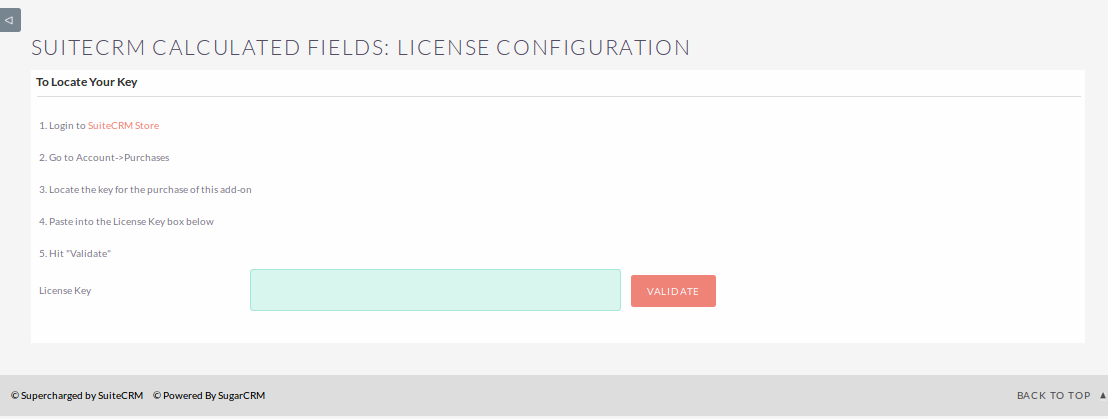Admin -> Repair -> Do 'Quick Repair & Rebuild'

• Studio -> 'Module' -> Add Fields -> Select 'Data Type' : Calculated Field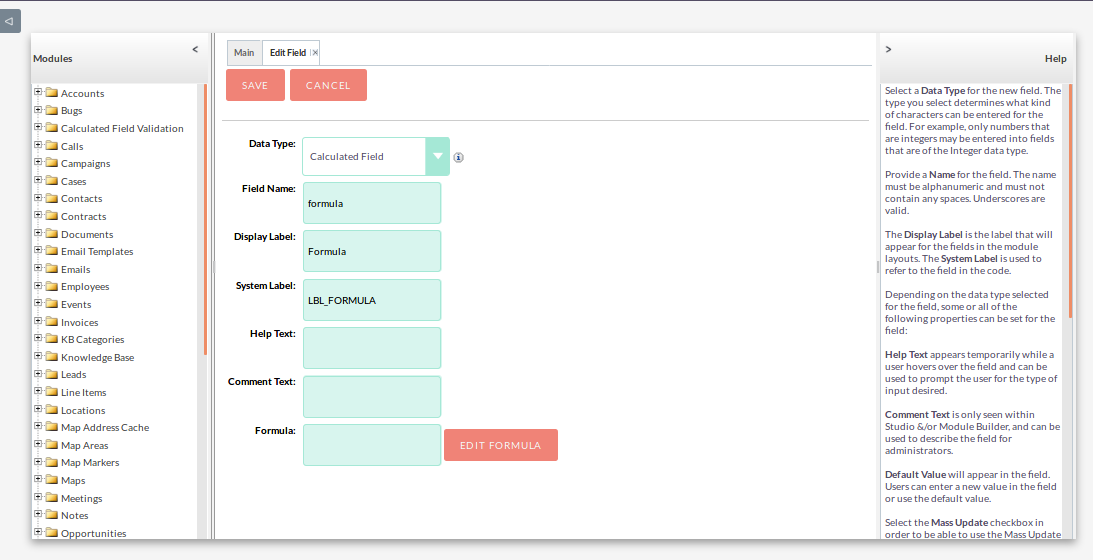• Click ‘Edit Formula’ to write your formula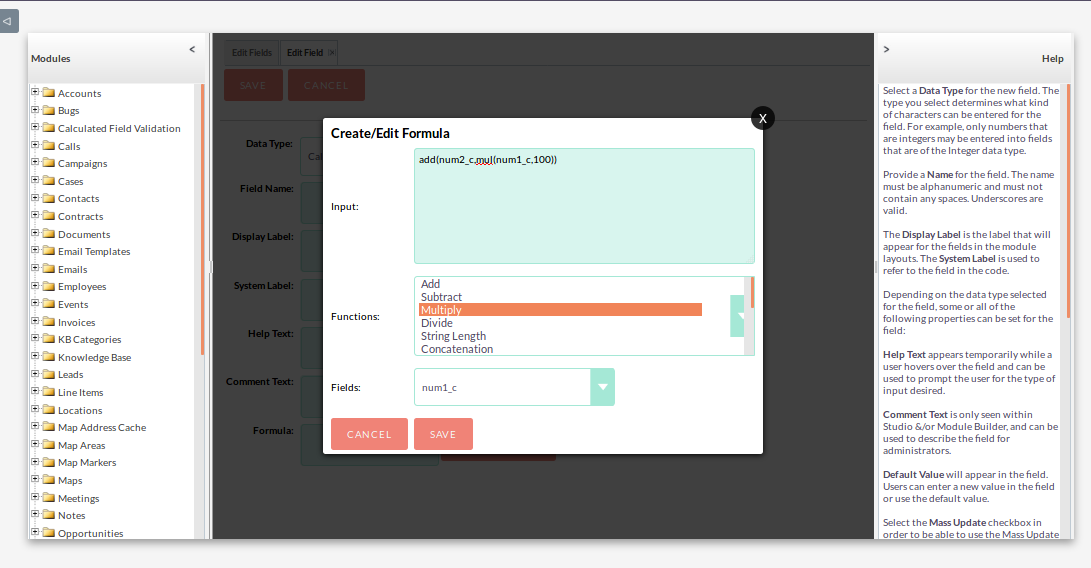Result of the above formula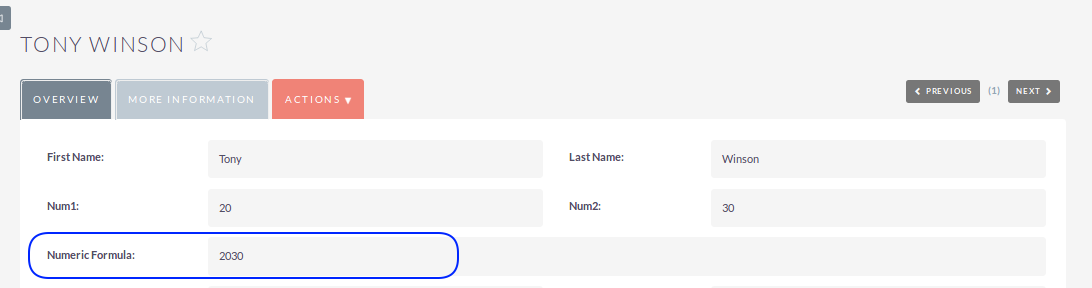• Calculate Percentage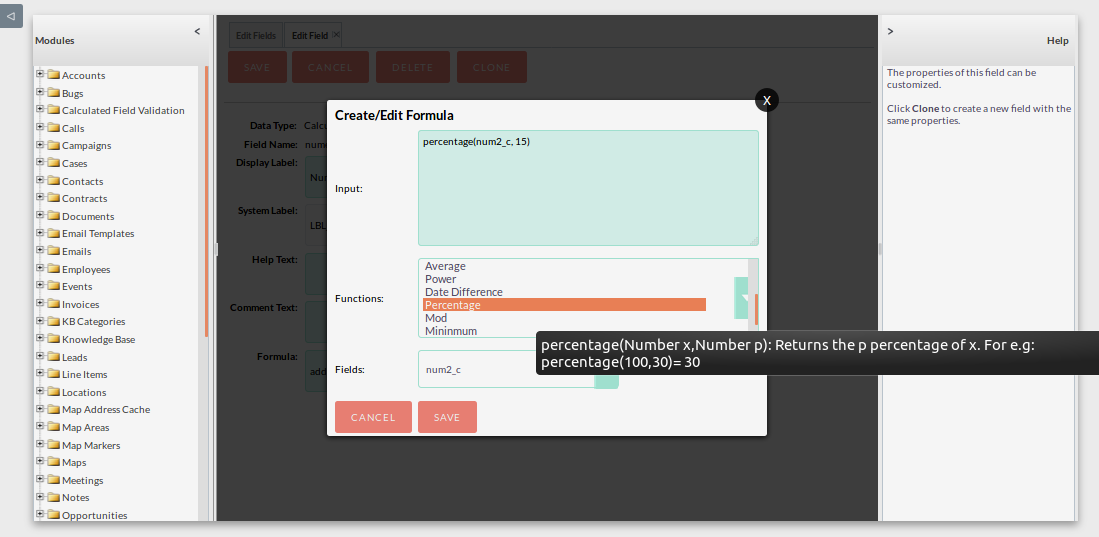Result of Percentage Formula• Calculate Date Difference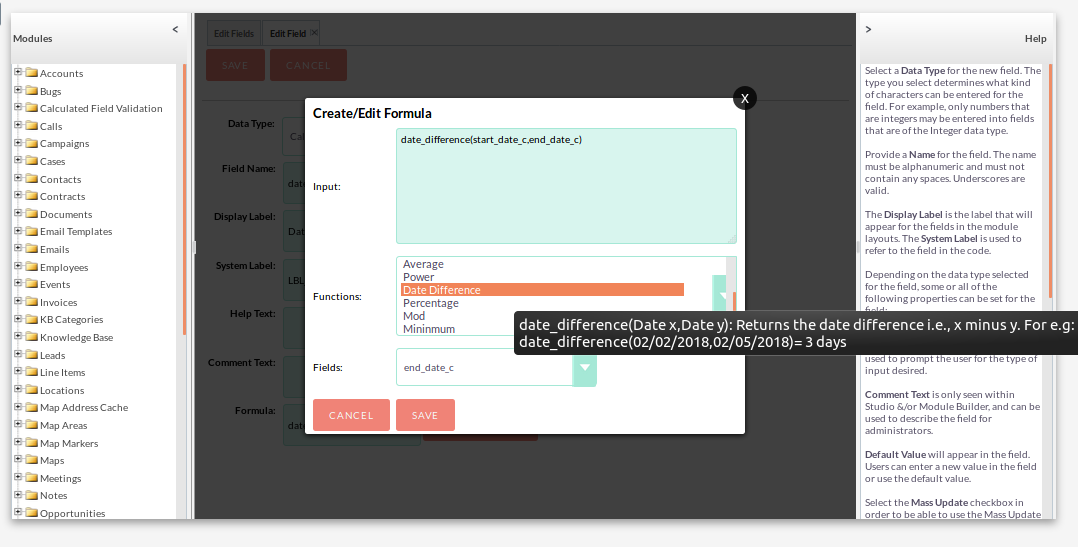Result of Date Difference• Concatenation Example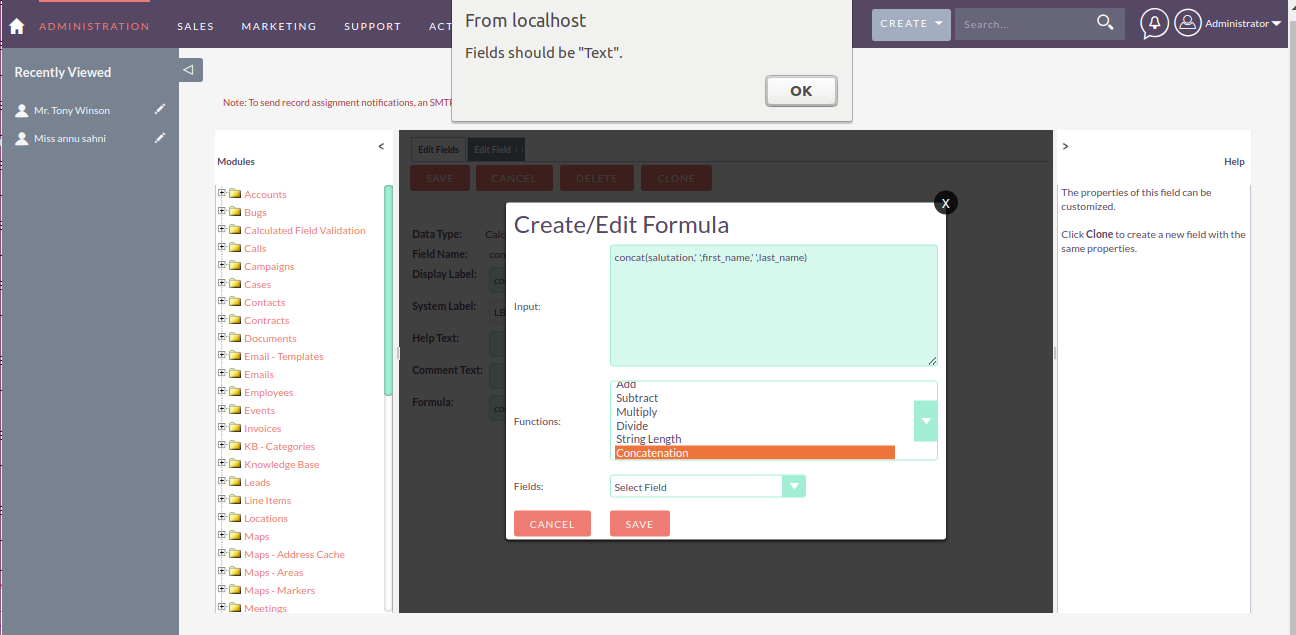Result of ConcatenationCount relationship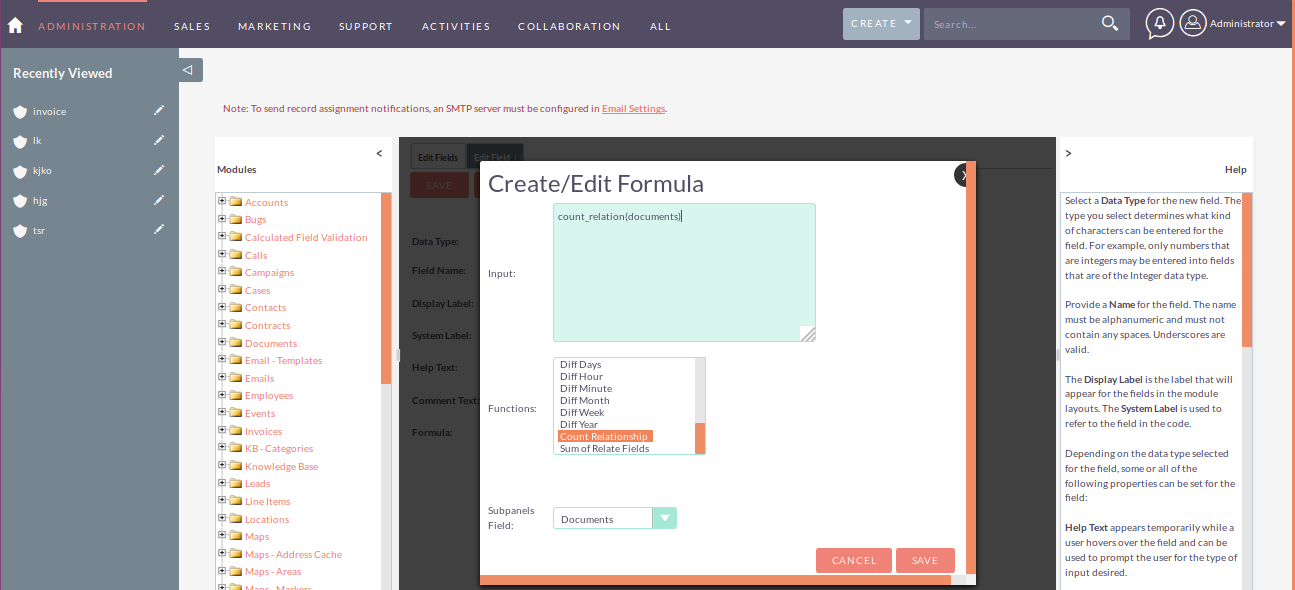Result of above formula-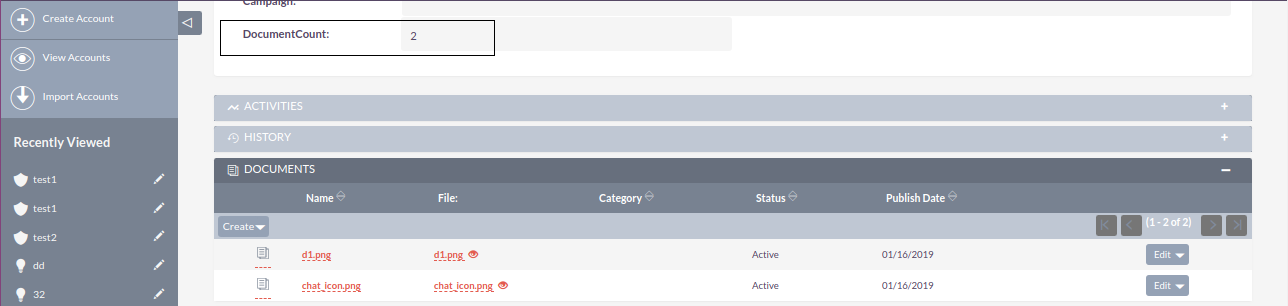Sum of relate FieldResult of above formula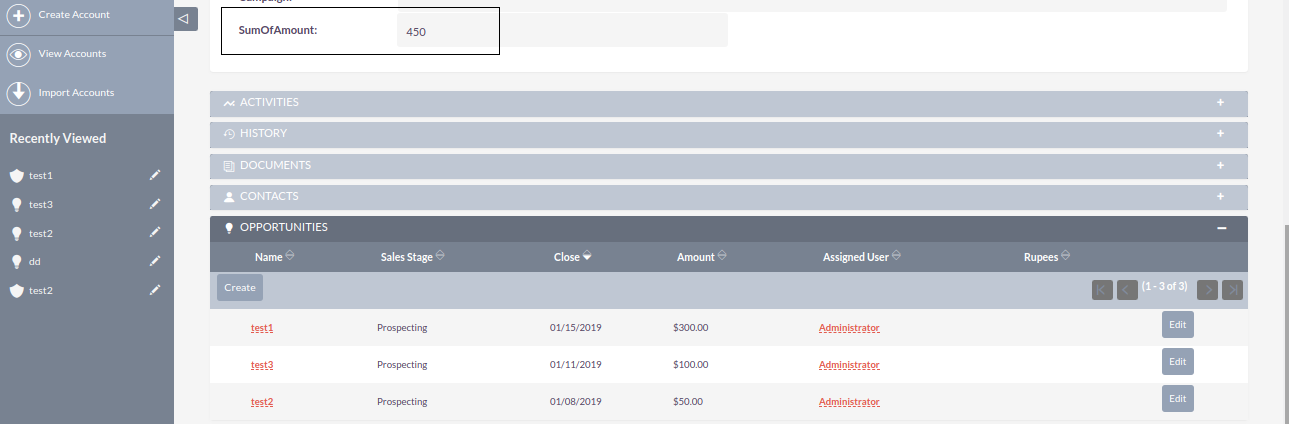### 4.Restrictions

• You have to enter proper syntax to retrieve the correct result. - If you open a function please close that. For e.g., add(mul(9,8),4 => Syntax Error ‘Close Parenthesis ’ Not found
• Use only ‘integer’ OR ‘decimal’ fields in Numeric operations
• Use only ‘date’ and ’datetime’ fields in Date Difference operations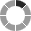Saving Comment...